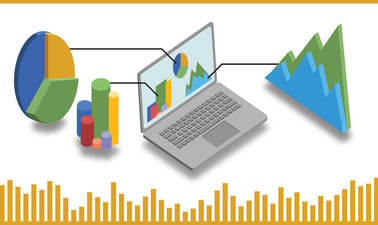# Applied Statistics with Python

Analyze data using statistical methods and technology.## There is one session available:

After a course session ends, it will be archived.
Estimated 16 weeks
8–10 hours per week
Self-paced
Free

This course helps prepare you for positions that require the analysis of large data sets, providing the statistics foundation you’ll need for data analysis.

You’ll learn how to model real-world applications using statistical methods. You’ll also gain skills to set up those applications in statistical frameworks, solve the statistical problems using technology and apply those results to answer real business questions. By leveraging the pandas and matplotlib python libraries, you’ll gain exposure to tools used in data analysis, visualization, and data science.

This course specifically explores inferential statistics - the science of applying statistical techniques to quantify and answer real-world data analysis questions.

The content covers three components – descriptive statistics and probability, hypothesis tests and confidence intervals and linear regression analysis.

Upon completing this course, you’ll be able to:

Formulate real-world data analysis questions as problems in statistical frameworks

• Explain how statistical concepts are applied in authentic situations

• Represent data numerically and visually

• Calculate and apply general and normal distribution probabilities

Analyze real-world problems using hypothesis tests and confidence interval techniques

• Construct hypothesis test and confidence interval models to solve problems

• Calculate hypothesis test and confidence interval results using technology

• Interpret the results of hypothesis test and confidence interval calculations

Analyze real-world problems using linear regression analysis techniques

• Construct linear regression models to solve problems

• Calculate linear regression results using technology

• Interpret the results of linear regression calculations

This is the second course in the introductory, undergraduate-level offering that makes up the larger Business Analytics Foundations MicroBachelors program. We recommend taking them in order, unless you have a background in these areas already and feel comfortable skipping ahead.

1. Scripting with Python - starting February 2021

2. Applied Statistics with Python - starting February 2021

NOTE : While this is generally a non-credit-bearing course, you may able to use this class with proper completion for 3 credits (1 course) toward a bachelor's degree at Southern New Hampshire University.

### At a glance

• Institution: SNHUx
• Subject: Data Analysis & Statistics
• Level: Intermediate
• Prerequisites:

This is the second course in the introductory, undergraduate-level offering that makes up the larger Business Analytics Foundations MicroBachelors program. We recommend taking them in order, unless you have a background in these areas already and feel comfortable skipping ahead.

1. Scripting with Python - starting February 2021

2. Applied Statistics with Python - starting February 2021

# What you'll learn

Skip What you'll learn
• Collect and classify data

• Summarize data using visual and numeric techniques

• Quantify answers to questions about estimating parameters using hypothesis test and confidence interval techniques

• Quantify answers to questions about correlation between variables using linear regression techniques